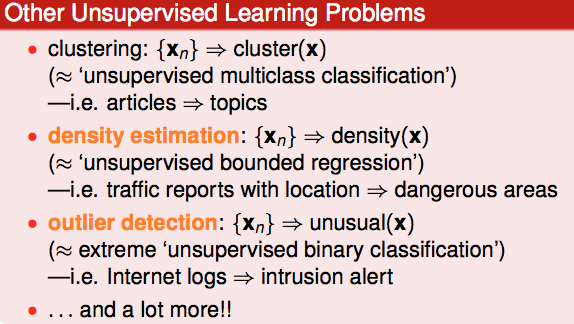## Learning with Different Output Space Y

### Classification

$$y=\{1,2,\dots,k\}$$ (Binary Classfication $$y=\{-1,+1\}$$)

### Regression

$$y=R$$

### Structured

$$y=Structures$$

\begin{aligned} &\underbrace{I}_{pronoun} \underbrace{love}_{verb} \text{ }\underbrace{ML}_{noun} \\\ &\text{sentence} \Rightarrow \text{structure(class of each word)} \\\ &y = \{PVN,PVP,NVN,PV,\dots\} \end{aligned}

ntence} \
&y = {PVN,PVP,NVN,PV,} \end{aligned}

## Learning with Different Data Label $$y_n$$

### Unsupervised## Learning With Different Protocol

1. batch：批量提供数据
2. online：在线分条序列提供数据
3. active：机器提问针对性获取 Label

## Learning With Different Input Space

1. Concrete Data：数据经过人工或者机器处理，X 的每一个维度都有一定的现实意义
2. Raw Data：未经处理的数据，如：图像的点阵。一般需要先提取特称(Concret Feature)
3. Abstract Data：抽象数据，如：用户 ID、音乐库 ID